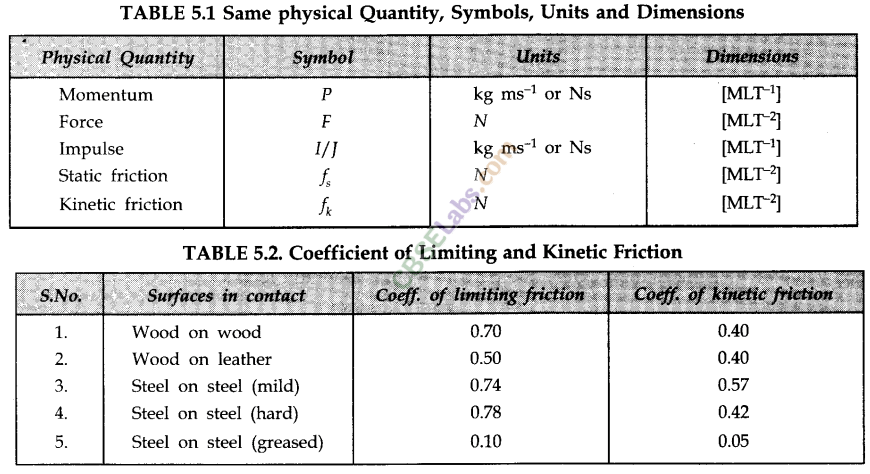Class 11 Physics Notes students can refer to the Law of Motion Class 11 Notes Physics Chapter 5 https://www.cbselabs.com/laws-motion-cbse-notes-class-11-physics/ Pdf here. They can also access the CBSE Class 11 Law of Motion Chapter 5 Notes while gearing up for their Board exams.

## Law of Motion Class 11 Notes Physics Chapter 5

Laws Of Motion Class 11 Chapter 5

• Dynamics is the branch of physics in which we study the motion of a body by taking into consideration the cause i.e., force which produces the motion.
• Force
Force is an external cause in the form of push or pull, which produces or tries to produce motion in a body at rest, or stops/tries to stop a moving body or changes/tries to change the direction of motion of the body.
• The inherent property, with which a body resists any change in its state of motion is called inertia. Heavier the body, the inertia is more and lighter the body, lesser the inertia.
• Law of inertia states that a body has the inability to change its state of rest or uniform motion (i.e., a motion with constant velocity) or direction of motion by itself.

Laws Of Motion Class 11 Notes Chapter 5

• Newton’s Laws of Motion
Law 1. A body will remain at rest or continue to move with uniform velocity unless an external force is applied to it.
First law of motion is also referred to as the ‘Law of inertia’. It defines inertia, force and inertial frame of reference.
I here is always a need of ‘frame of reference’ to describe and understand the motion of particle, lhc simplest ‘frame of reference’ used are known as the inertial frames.
A frame of referent, e is known as an inertial frame it, within it, all accelerations of any particle are caused by the action of ‘real forces’ on that particle.
When we talk about accelerations produced by ‘fictitious’ or ‘pseudo’ forces, the frame of reference is a non-inertial one.
Law 2. When an external force is applied to a body of constant mass the force produces an acceleration, which is directly proportional to the force and inversely proportional to the mass of the body.Law 3. “To every action there is equal and opposite reaction force”. When a body A exerts a force on another body B, B exerts an equal and opposite force on A.
• Linear Momentum
The linear momentum of a body is defined as the product of the mass of the body and its velocity.Class 11 Physics Chapter 5 Notes

• Impulse
Forces acting for short duration are called impulsive forces. Impulse is defined as the product of force and the small time interval for which it acts. It is given by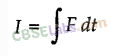Impulse of a force is a vector quantity and its SI unit is 1 Nm.
— If force of an impulse is changing with time, then the impulse is measured by finding the area bound by force-time graph for that force.
— Impulse of a force for a given time is equal to the total change in momentum of the body during the given time. Thus, we have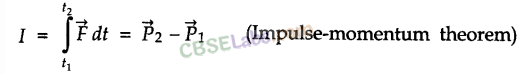• Law of Conservation of Momentum
The total momentum of an isolated system of particles is conserved.
In other words, when no external force is applied to the system, its total momentum remains constant.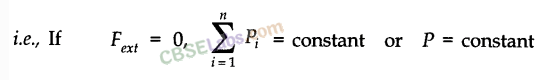• Recoiling of a gun, flight of rockets and jet planes are some simple applications of the law of conservation of linear momentum.Law Of Motion Class 11 Chapter 5

• Concurrent Forces and Equilibrium
“A group of forces which are acting at one point are called concurrent forces.”
Concurrent forces are said to be in equilibrium if there is no change in the position of rest or the state of uniform motion of the body on which these concurrent forces are acting.
For concurrent forces to be in equilibrium, their resultant force must be zero. In case of three concurrent forces acting in a plane, the body will be in equilibrium if these three forces may be completely represented by three sides of a triangle taken in order. If number of concurrent forces is more than three, then these forces must be represented by sides of a closed polygon in order for equilibrium.Laws Of Motion Notes Chapter 5

• Commonly Used Forces
(i) Weight of a body. It is the force with which earth attracts a body towards its centre. If M is mass of body and g is acceleration due to gravity, weight of the body is Mg in vertically downward direction.
(ii) Normal Force. If two bodies are in contact a contact force arises, if the surface is smooth the direction of force is normal to the plane of contact. We call this force as Normal force.Example. Let us consider a book resting on the table. It is acted upon by its weight in vertically downward direction and is at rest. It means there is another force acting on the block in opposite direction, which balances its weight. This force is provided by the table and we call it as normal force.
(iii) Tension in string. Suppose a block is hanging from a string. Weight of the block is acting vertically downward but it is not moving, hence its weight is balanced by a force due to string. This force is called ‘Tension in string’. Tension is a force in a stretched string. Its direction is taken along the string and away from the body under consideration.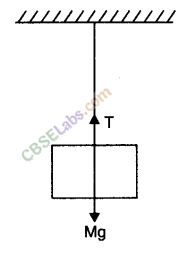Laws Of Motion Class 11 Notes Pdf Chapter 5

• Simple Pulley
Consider two bodies of masses m1 and m2 tied at the ends of an in extensible string, which passes over a light and friction less pulley. Let m1 > m2. The heavier body will move downwards and the lighter will move upwards. Let a be the common acceleration of the system of two bodies, which is given by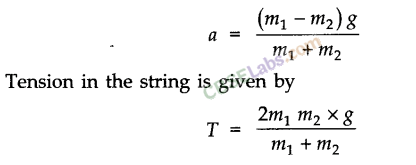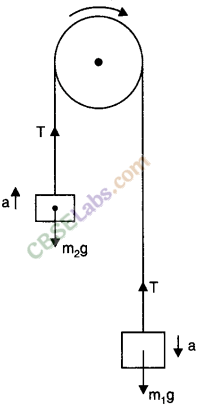Class 11 Laws Of Motion Notes Chapter 5

• Apparent Weight and Actual Weight
— ‘Apparent weight’ of a body is equal to its ‘actual weight’ if the body is either in a state of rest or in a state of uniform motion.
— Apparent weight of a body for vertically upward accelerated motion is given as
Apparent weight =Actual weight + Ma = M (g + a)
— Apparent weight of a body for vertically downward accelerated motion is given as
Apparent weight = Actual weight Ma = M (g – a).
• Friction
The opposition to any relative motion between two surfaces in contact is referred to as friction. It arises because of the ‘inter meshing’ of the surface irregularities of the two surfaces in contact.
• Static and Dynamic (Kinetic) Friction
The frictional forces between two surfaces in contact (i) before and (ii) after a relative motion between them has started, are referred to as static and dynamic friction respectively. Static friction is always a little more than dynamic friction.
The magnitude of kinetic frictional force is also proportional to normal force.Law Of Motion Class 11 Notes Chapter 5

• Limiting Frictional Force
This frictional force acts when body is about to move. This is the maximum frictional force that can exist at the contact surface. We calculate its value using laws of friction.
Laws of Friction:
(i) The magnitude of limiting frictional force is proportional to the normal force at the contact surface.(ii) The magnitude of limiting frictional force is independent of area of contact between the surfaces.
• Coefficient of Friction
The coefficient of friction (μ) between two surfaces is the ratio of their limiting frictional force to the normal force between them, i.e.,Chapter 5 Physics Class 11 Notes

• Angle of Friction
It is the angle which the resultant of the force of limiting friction F and the normal reaction R makes with the direction of the normal reaction. If θ is the angle of friction, we have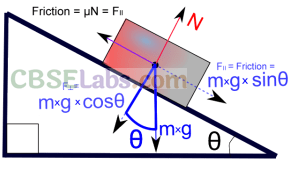• Angle of Repose
Angle of repose (α) is the angle of an inclined plane with the horizontal at which a body placed over it just begins to slide down without any acceleration. Angle of repose is given by α = tan-1 (μ)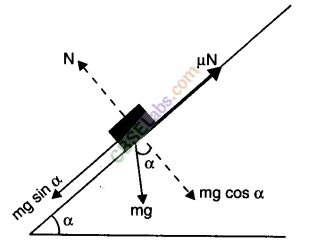Ch 5 Physics Class 11 Notes

• Motion on a Rough Inclined Plane
Suppose a motion up the plane takes place under the action of pull P acting parallel to the plane.
• Centripetal Force
Centripetal force is the force required to move a body uniformly in a circle. This force acts along the radius and towards the centre of the circle. It is given by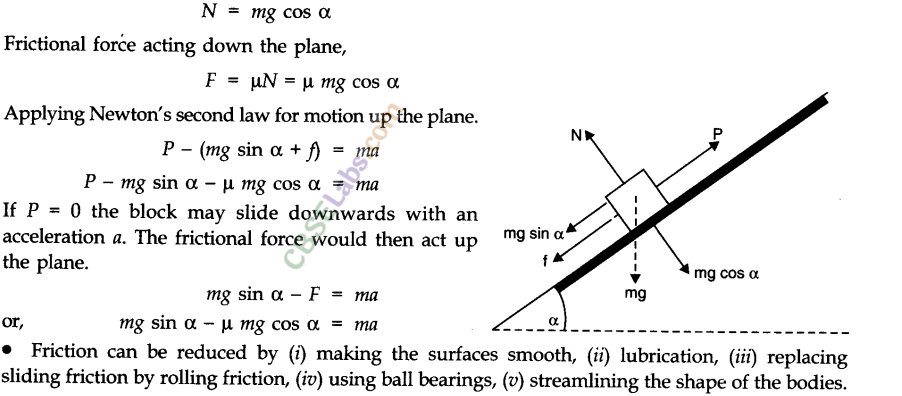where, v is the linear velocity, r is the radius of circular path and ω is the angular velocity of the body.
• Centrifugal Force
Centrifugal force is a force that arises when a body is moving actually along a circular path, by virtue of tendency of the body to regain its natural straight line path.
The magnitude of centrifugal force is same as that of centripetal force.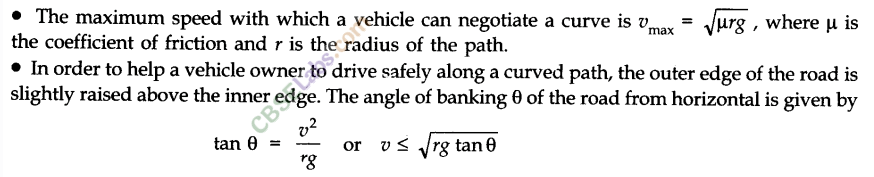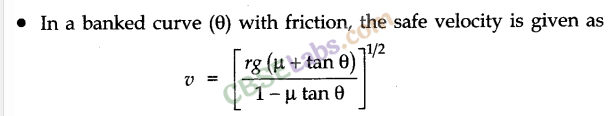• Motion in a Vertical Circle
The motion of a particle in a horizontal circle is different from the motion in vertical circle. In horizontal circle, the motion is not effected by the acceleration due to gravity (g) whereas in the motion of vertical circle, the motion is not effected by the acceleration due to gravity (g) whereas in the motion of vertical circle, the value of ‘g’ plays an important role, the motion in this case does not remain uniform. When the particle move up from its lowest position P, its speed continuously decreases till it reaches the highest point of its circular path. This is due to the work done against the force of gravity. When the particle moves down the circle, its speed would keep on increasing.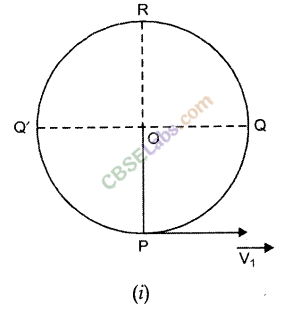Let us consider a particle moving in a circular vertical path of radius V and centre o tide with a string. L be the instantaneous position of the particle such that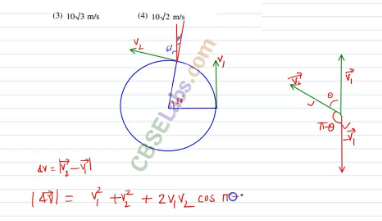Here the following forces act on the particle of mass ‘m’.
(i) Its weight = mg (verticaly downwards).
(ii) The tension in the string T along LO.We can take the horizontal direction at the lowest point ‘p’ as the position of zero gravitational potential energy. Now as per the principle of conservation of energy,From this relation, we can calculate the tension in the string at the lowest point P, mid-way point and at the highest position of the moving particle.
Case (i) : At the lowest point P, θ = 0°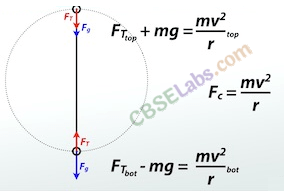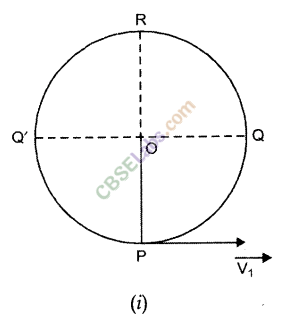When the particle completes its motion along the vertical circle, it is referred to as “Looping the Loop” for this the minimum speed at the lowest position must be √5gr
• IMPORTANT TABLES